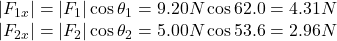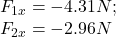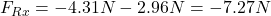Question

Two forces, F⃗ 1F→1F_1_vec and F⃗ 2F→2F_2_vec, act at a point. F⃗ 1F→1F_1_vec has a magnitude of 9.20 NN and is directed at an angle of 62.0 ∘∘ above the negative x axis in the second quadrant. F⃗ 2F→2F_2_vec has a magnitude of 5.00 NN and is directed at an angle of 53.6 ∘∘ below the negative x axis in the third quadrant. Part A What is the x component of the resultant force?

1.quangkhai

The x component of the resultant force is -7.27N.

Explanation:

To obtain the x component of the resultant force, first we have to know the x components of the other forces. To do this, we just have to do some trigonometry:Since both vectors are in the left side of the y-axis, they have a negative x component. So:Finally, we sum both components to obtain the component of the resultant force:In words, the x component of the resultant force is -7.27N.